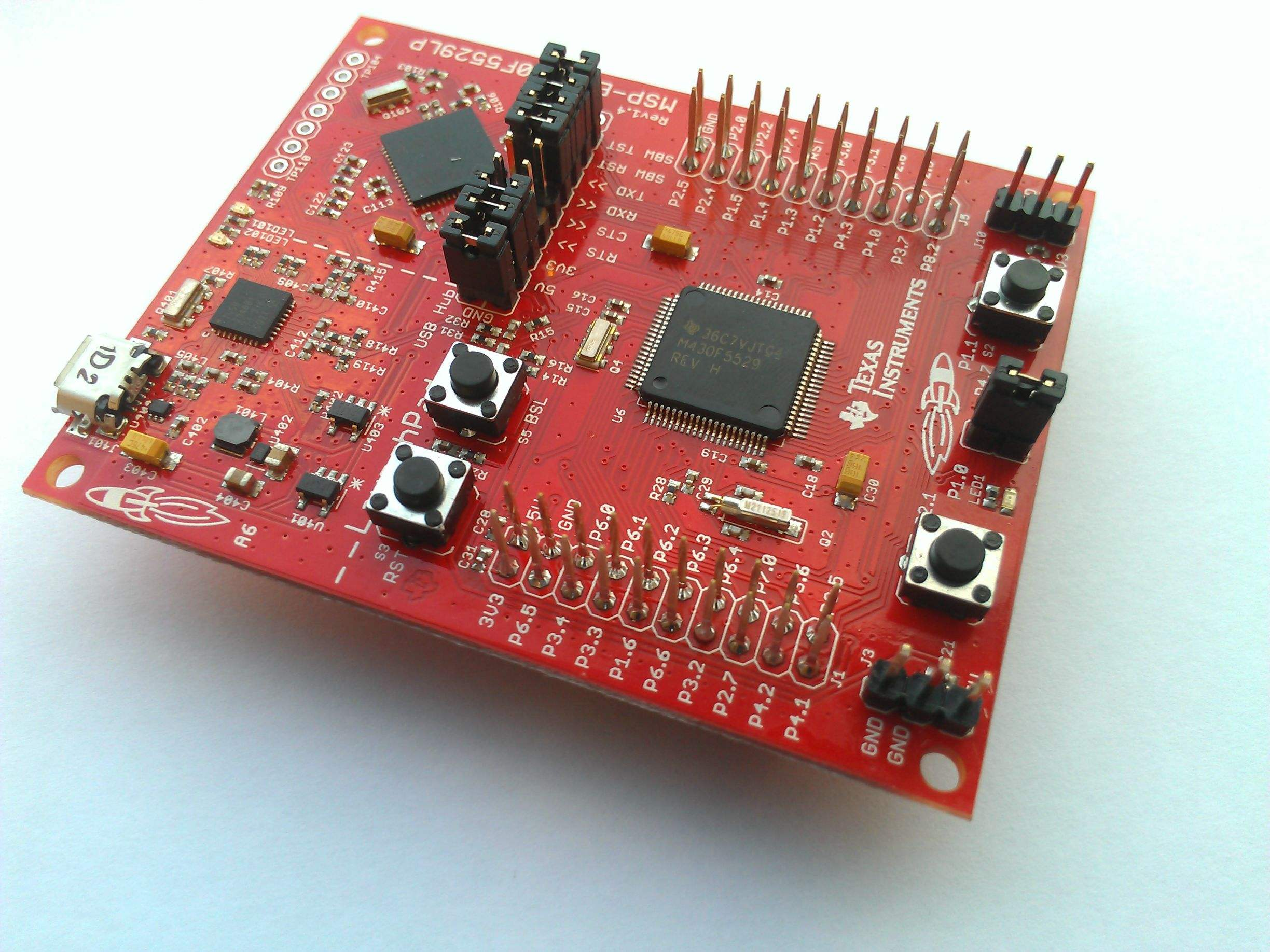## 用汇编语言实现奇偶校验以及海明码

2880 0 2018年11月2日

0 0 投票数

1. 每次取出原数据的最低位，这个可以通过原始数据和 $1$ 做位与运算得到
2. 将取出的最低位与校验位做异或，得到的结果保留到校验位
3. 重复上面两个步骤，直到原数据的每一位都被取出来过
4. 这时候校验位的值相当于记录了 $1$ 的个数
5. 由于是奇校验，所以对校验位再做一次和 $1$ 的异或，得到校验位的值
6. 将原数据左移 $1$ 位，然后把校验位的值填入最低位
7. 可以通过将左移后的值与校验位做位与运算完成上面这个操作
8. 最后得到的就是答案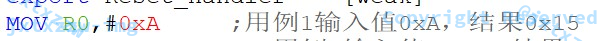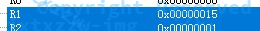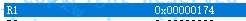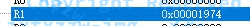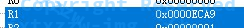$$r_0={I_7}\oplus{I_5}\oplus{I_4}\oplus{I_2}\oplus{I_1} \ r_1={I_7}\oplus{I_6}\oplus{I_4}\oplus{I_3}\oplus{I_1} \ r_2={I_8}\oplus{I_4}\oplus{I_3}\oplus{I_2} \ r_3={I_8}\oplus{I_7}\oplus{I_6}\oplus{I_5}$$

$$s_0={I_7}\oplus{I_5}\oplus{I_4}\oplus{I_2}\oplus{I_1}\oplus{r_0} \ s_1={I_7}\oplus{I_6}\oplus{I_4}\oplus{I_3}\oplus{I_1}\oplus{r_1} \ s_2={I_8}\oplus{I_4}\oplus{I_3}\oplus{I_2}\oplus{r_2} \ s_3={I_8}\oplus{I_7}\oplus{I_6}\oplus{I_5}\oplus{r_3}$$

1. 输入的原数据是不知道长度的，如何知道有多少位 $K$ ？
2. 如何根据公式 $\displaystyle 2^R \geqslant K + R + 1$ 确定 $R$ 的值？
3. 确定了 $R$ 的值以后，如何知道是哪几位是校验位？
4. 计算海明码的时候，对于第 $i$ 位的数据，如何确定要和哪个或者哪几个校验位做异或？
5. 异或的结果如何保存？
6. 如何随便修改一位？
7. 重新校验的时候，对于第 $i$ 位的数据，如何跳过校验位？
8. 根据校验后的结果，如何确定第几位出错了？
9. 修改后的数据，如何还原为原数据？

1. 输入的原数据是不知道长度的，如何知道有多少位 $K$ ？
这个比较简单，将一个数循环右移多少次以后得到 $0$，就是这个数有多少位，于是可以得到 $K$ 的值
2. 如何根据公式 $\displaystyle 2^R \geqslant K + R + 1$ 确定 $R$ 的值？

由于 $K$ 最少是 $1$、最多是 $32$

所以由公式 $\displaystyle 2^R \geqslant K + R + 1$ 显然有，$2 \leqslant R \leqslant 6$

那么，就可以令 $R$ 为 $2$，每次判断 $\displaystyle 2^R \geqslant K + R + 1$ 是否成立，如果成立，则找到 $R$ 的值，否则$R$ 加 $1$

最后必然会有一个 $2 \leqslant R \leqslant 6$ 满足 $\displaystyle 2^R \geqslant K + R + 1$

在实际操作的过程中，可以用一个寄存器 $R_1$ 记录 $2^R$，用一个寄存器 $R_2$ 记录 $K+R+1$，如果$R_1 \lt R_2$，那么 $R_1$ 左移一位，$R_2$ 加 $1$

CMP R4,R5
BGE TODO
LSL R4,#1 ;R+1，2^{R+1}=R4<<1
B LOOPR
3. 确定了 $R$ 的值以后，如何知道是哪几位是校验位？
校验位一定是第 $1,2,4,8,\ldots$ 位

所以可以令寄存器 $R_1$ 从 $1$ 开始，每次左移 $1$ 位，直到超过了 $K+R$ 位

当已知了 $R$，那么 $N=K+R$ 就是一个常数了

4. 计算海明码的时候，对于第 $i$ 位的数据，如何确定要和哪个或者哪几个校验位做异或？

$i$ 是下标，所以需要有一个寄存器作为 $INDEX$，从 $N$ 遍历到 $0$，每次取出第 $INDEX$ 位的数据

记取出的数据为 $X$，那么 $X$ 就是要参与异或运算的，$X=0$ 或者 $X=1$

而 $INDEX=i$ 是下标，下面将这个十进制的下标转化为二进制

例如对于 $i=6$，其二进制表示是 $110$，说明需要对第 $3$ 和第 $2$ 个校验位做异或，例如对于 $i=5$，其二进制表示是 $101$，说明需要对第 $3$ 和第 $1$ 个校验位做异或

那么，将一个数从十进制转化为二进制，就是除 $2$ 取余、除 $2$ 取余依次循环

当取出当前位为 $0$ 的时候，说明不需要对该位进行校验异或

当取出当前位为 $0$ 的时候，说明需要对该位进行校验异或，此时，应该将刚才取出的 $X$ 的值，与当前的校验位做异或

EOR R8,R8,R1 ;R1是当前位的值，R8是对应校验位的值，做异或

由于除 $2$ 取余这个过程每次取出的是最低位，所以令 $R_1$ 表示当前校验位的位置的话，$R_1$ 的初始值为 $1$

之后每取出一位，如果这一位是 $1$，就令 $X$ 与 $R_1$ 表示的校验位做异或，然后 $R_1$ 左移一位，如果这一位是 $0$，就直接 $R_1$ 左移一位

显然的，当 $INDEX=i$ 右移到 $0$ 的时候， $R_1$ 也刚好超过了 $N=K+R$，两者的结束条件一致

CMP R7,#0
BEQ ITERNEXT ;这一位的二进制全处理完了，遍历下一个数字
TST R7,#1
BEQ SKIPXOR ;说明这一位二进制是0，那么不用与对应的校验位做异或

当这一位的校验结束以后，遍历 $INDEX$，即执行 $INDEX=INDEX-1$，然后重置 $R_1$ 为 $1$

MOV R4,#1
SUB R2,R2,#1 ;INDEX--
CMP R2,#0 ;INDEX到0说明全部做完了

特别的，当 $INDEX$ 遇到校验位的时候，应该跳过该校验位，不做异或，如何知道是哪几位是校验位见上一条

CMP R2,R5
BNE BEGINITER ;R2不等于R5，说明这一位不是校验位，就开始处理
;这一位是校验位，那么跳过，继续遍历
LSR R5,#1 ;校验位除2
B ITERNEXT
LSL R4,#1 ;校验位从1、2、4……移动
LSR R7,#1 ;右移一位，开始看下一位要不要做异或
5. 异或的结果如何保存？
原数据存哪，异或的结果就存哪，我是存内存的
6. 如何随便修改一位？

因为汇编语言没有产生随机数的功能，所以必须指定一位，考虑到 $K$ 最小是 $1$，我就修改了第 $3$ 位

7. 重新校验的时候，对于第 $i$ 位的数据，如何跳过校验位？

与上面类似的，有一个寄存器 $R_1$ 从 $1$ 开始，每次左移 $1$ 位，即 $R_1$ 表示下一个校验位的位置

当满足 $INDEX=R_1$ 的时候，说明这一位是校验位，跳过，然后 $R_1$ 左移 $1$ 位

8. 根据校验后的结果，如何确定第几位出错了？

校验的过程与计算海明码的过程是类似的

$i$ 是下标，所以需要有一个寄存器作为 $INDEX$，从 $N$ 遍历到 $0$，每次取出第 $INDEX$ 位的数据

记取出的数据为 $X$，那么 $X$ 就是要参与异或运算的，$X=0$ 或者 $X=1$

令 $R_1$ 表示当前校验位的位置，对 $INDEX$ 循环右移，每取出一位，如果这一位是 $1$，就令 $X$ 与 $R_1$ 表示的校验位做异或，然后 $R_1$ 左移一位，如果这一位是 $0$，就直接 $R_1$ 左移一位

特别的，当 $INDEX$ 遇到校验位的时候，应该跳过该校验位，不做异或

之后，所有校验位的值都是校验后的值，依次遍历所有的校验位，乘 $2$ 累加，将二进制转化为十进制，求出的就是第几位数据出错了

LOOPPRODSUM
CMP R4,#0
BEQ FINDWHO ; 说明已经算完了全部的校验位
LSL R5,#1 ;左移1位
SUB R6,R3,R4
SUB R6,R6,#1 ;R6表示内存的偏移量
LDRB R7,[R0,R6] ;R7是当前要处理的校验位的值
LSR R4,#1 ;校验位除2
B LOOPPRODSUM ;二进制转十进制

至此，已经求出了出错的位在哪里

然后取反，还原得到原数据

9. 修改后的数据，如何还原为原数据？

二进制转十进制，乘 $2$ 累加

特别的，需要跳过校验位

LOOPBINTDEC
SUB R4,R4,#1 ;R4--
CMP R4,#0
BEQ EXIT
CMP R2,R4
BEQ BINTDECNEXT ;这一位是校验位，跳过
LSL R5,#1 ;乘2
SUB R6,R3,R4
SUB R6,R6,#1 ;R6表示内存的偏移量
LDRB R7,[R0,R6] ;从内存取出一个数
B LOOPBINTDEC
BINTDECNEXT
LSR R2,#1 ;下一个校验位的位置，除2
B LOOPBINTDEC
EXIT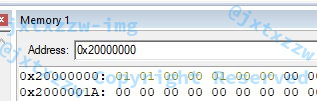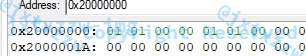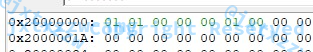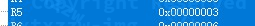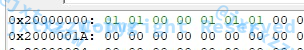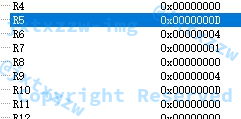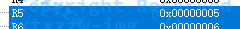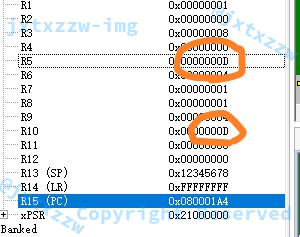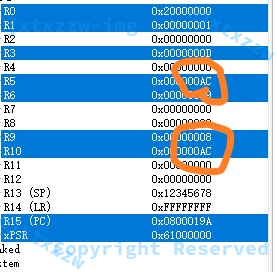$1$ 位也行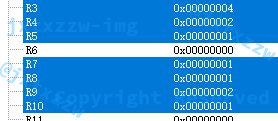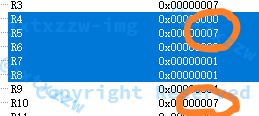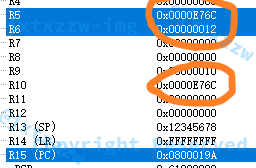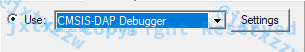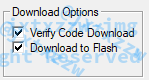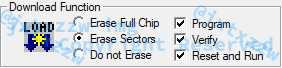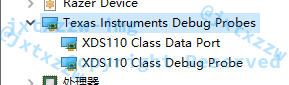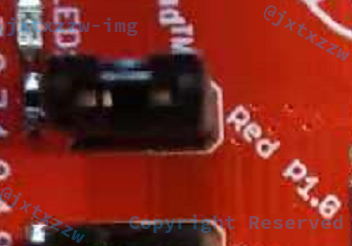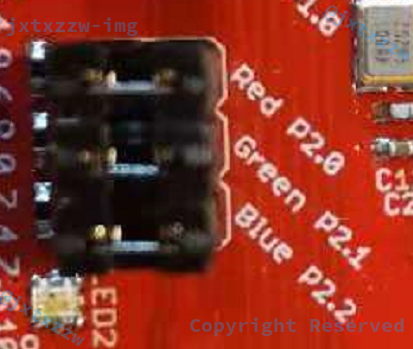GPIO_PORT_P2,
GPIO_PIN1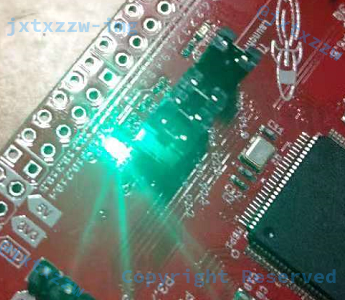AREA Reset,DATA,READONLY
DCD 0X12345678
DCD   Reset_Handler
Reset_Handler  proc
export Reset_Handler    [weak]
;MOV R0,#0xA     ;用例1输入值0xA，结果0x15
;MOV R0,#0xBA     ;用例2输入值0xBA，结果0x174
;MOV R0,#0xCBA   ;用例3输入值0xCBA，结果0x1974
MOV R0,#0x7654  ;用例4输入值0x7654，结果0xECA9
MOV R1,R0        ;R1存储结果
MOV R2,#0        ;R2存储1的个数
MOV R3,#0        ;R3作为临时寄存器，最多只能使用R0、R1、R2、R3及xPSR寄存器
;//---------------请在以下空白区域内编写代码------------//
MOV R1,R0 ;R1存储结果，先把原始数据保留下来
LOOP
CMP R0,#0 ;循环右移，每次取出最低位，直到取完所有位数
BEQ BRK
AND R3,R0,#1 ;数与1做位与，得到的就是最低位的值，保留到R3中
LSR R0,#1 ;R0循环右移
EOR R2,R3,R2 ;R2是异或运算的结果，R3是最低位，做异或
B LOOP
BRK
EOR R2,R2,#1 ;因为奇校验，所以再做一次异或，该值就是校验位的值
LSL R1,#1 ;R1是原始数据，左移1位
ORR R1,R1,R2 ;然后和校验位做位或，就是把校验位放到最低位，得到的结果就是最后的值
;//---------------请在以上空白区域内编写代码------------//
;最终结果存入R1
NOP         ;检查时，请直接跳转至此
ENDP

; 海明码
DCD 0x12345678
DCD   Reset_Handler
Reset_Handler  proc
export Reset_Handler    [weak]
MOV R0,#0x20000000 ;内存基址
;MOV R1,#0xAC ;8位
MOV R1,#0xD ;4位
;MOV R1,#0x1 ;1位
;MOV R1,#0x7 ;3位
MOV R10,R1 ;把R1的值保留到R10，是为了最后检查是不是正确还原
MOV R3,#0 ;R3计算数据有多少位
MOV R2,R1 ;保留原数据
CALCLEN
CMP R2,#0 ;循环右移直到0，计算有多少位
BEQ BEGINCALCR
LSR R2,#1
B CALCLEN
BEGINCALCR
MOV R4,#2 ;R4计算需要多少位校验位，显然最少是2位，从2开始尝试，R4=2^{R}
MOV R5,#1
MOV R6,#1
LSL R4,R6,R4 ;R4=2^{R}=1<<R=R6<<R4
LOOPR
CMP R4,R5
BGE BEGINSTORE
LSL R4,#1 ;R+1，2^{R+1}=R4<<1
B LOOPR
BEGINSTORE
;SUB R3,R5,#1 ;R=(K+R+1)-1-K=R5-1-R3，N=K+R=R3+R5-1-R3=R5-1
MOV R3,R5 ;R=(K+R+1)-1-K=R5-1-R3，N=K+R=R3+R5-1-R3=R5-1
; 至此，准备工作完成，R0是基址，R1是数据，R3是N=K+R，其余寄存器已经没用了
MOV R4,#1 ;R4表示INDEX，即当前在处理第几位，一开始处理第1位，之后每次加1
MOV R5,#1 ;R5表示下一个校验位要放在那里，一开始放在1，之后每次乘2
LOOPSTORE
CMP R4,R3
BEQ BEGINHAMMING ;INDEX=N，说明处理完了，BREAK
AND R2,R1,#1 ;R1是尚未处理的数据，与1做位与，取出最低位放在R2
LSR R1,#1 ;R1>>1，处理了最后一位，剩下的右移
RECMPOBLIGATE
CMP R4,R5
BNE STORESKIPOBLIGATE
;R4=R5说明这一位是校验位，留空
MOV R4,#0 ;借用寄存器R4，存个0
SUB R6,R3,R5 ;R6=R3-R4=R3-R5=N-INDEX，是内存的偏移量，因R4被借用了，R5=R4
SUB R6,R6,#1
STRB R4,[R0,R6] ;这一位是校验位，用0填充
LSL R5,#1 ;下一个校验位的位置是乘2
B RECMPOBLIGATE
STORESKIPOBLIGATE
;否则说明这一位不是校验位，要填在这一位
SUB R6,R3,R4 ;R6=R3-R4=N-INDEX，是内存的偏移量
SUB R6,R6,#1
STRB R2,[R0,R6] ;用字节存储，存到R0基址偏移R6的地方
B LOOPSTORE
BEGINHAMMING ;开始计算海明码
;至此，R0表示基址，R3表示N=K+R，R5表示下一个校验位在第几位，其余寄存器已经没用了
LSR R5,#1 ;先把表示校验位的标志号退回来
MOV R9,R5 ;把最高位的校验位所在的位置记下来，省的后面再算（虽然后面也可以算得出来）
MOV R2,R3 ;R2=INDEX，表示当前遍历到第几位
ITERNEXT
SUB R2,R2,#1 ;INDEX--
CMP R2,#0 ;INDEX到0说明全部做完了
BEQ ENDHAMMING
CMP R2,R5
BNE BEGINITER ;R2不等于R5，说明这一位不是校验位，就开始处理
;这一位是校验位，那么跳过，继续遍历
LSR R5,#1 ;校验位除2
B ITERNEXT
BEGINITER
MOV R4,#1 ;表示当前在做哪一个校验位，每一个数字肯定从1开始检查
SUB R6,R3,R2
SUB R6,R6,#1 ;R6表示内存的偏移量
LDRB R1,[R0,R6] ;从内存R0偏移R6取出一个字节到寄存器R1，R1表示当前处理的值
MOV R7,R2 ;R2是INDEX，不能动，但是要取出R2的二进制，所以只能复制一份
LOOPXOR
CMP R7,#0
BEQ ITERNEXT ;这一位的二进制全处理完了，遍历下一个数字
TST R7,#1
BEQ SKIPXOR ;说明这一位二进制是0，那么不用与对应的校验位做异或
SUB R6,R3,R4
SUB R6,R6,#1 ;R6表示内存的偏移量
LDRB R8,[R0,R6] ;R8是当前要处理的校验位的值
EOR R8,R8,R1 ;R1是当前位的值，R8是对应校验位的值，做异或
STRB R8,[R0,R6] ;存回去
SKIPXOR
LSL R4,#1 ;校验位从1、2、4……移动
LSR R7,#1 ;右移一位，开始看下一位要不要做异或
B LOOPXOR
ENDHAMMING
;至此，海明码已经求完，结果见内存
;R0表示基址，R3表示N=K+R，其余寄存器已经没用了
MODIFY
;下面随机修改一个值，不妨修改第3位为他的取反
SUB R6,R3,#3
SUB R6,R6,#1
LDRB R1,[R0,R6] ;这里以修改掉第3位为例
EOR R1,R1,#1 ;取反，和1做异或
STRB R1,[R0,R6]
BEGINCHECK ;开始计算校验海明码
MOV R5,#1 ;下一个校验位在几号
MOV R2,#0 ;R2=INDEX，表示当前遍历到第几位
CHECKNEXT
CMP R2,R3 ;INDEX到N+1说明全部做完了
BEQ ENDCHECK
CMP R2,R5
BNE CHECKITER ;R2不等于R5，说明这一位不是校验位，就开始处理
;这一位是校验位，那么跳过，继续遍历
LSL R5,#1 ;校验位乘2
B CHECKNEXT
CHECKITER
MOV R4,#1 ;表示当前在做哪一个校验位，每一个数字肯定从1开始检查
SUB R6,R3,R2
SUB R6,R6,#1 ;R6表示内存的偏移量
LDRB R1,[R0,R6] ;从内存R0偏移R6取出一个字节到寄存器R1，R1表示当前处理的值
MOV R7,R2 ;R2是INDEX，不能动，但是要取出R2的二进制，所以只能复制一份
LOOPCHECKXOR
CMP R7,#0
BEQ CHECKNEXT ;这一位的二进制全处理完了，遍历下一个数字
TST R7,#1
BEQ SKIPCHECKXOR ;说明这一位二进制是0，那么不用与对应的校验位做异或
SUB R6,R3,R4
SUB R6,R6,#1 ;R6表示内存的偏移量
LDRB R8,[R0,R6] ;R8是当前要处理的校验位的值
EOR R8,R8,R1 ;R1是当前位的值，R8是对应校验位的值，做异或
STRB R8,[R0,R6] ;存回去
SKIPCHECKXOR
LSL R4,#1 ;校验位从1、2、4……移动
LSR R7,#1 ;右移一位，开始看下一位要不要做异或
B LOOPCHECKXOR
ENDCHECK
;至此，海明码校验结果已经保存在各个校验位，R0是基址，R3是一共多少位，其余寄存器已经没用了
;下面开始找哪一位出错了
MOV R4,R9 ;从最左边的校验位开始
MOV R5,#0 ;R5表示S，计算哪一位出错了
LOOPPRODSUM
CMP R4,#0
BEQ FINDWHO ; 说明已经算完了全部的校验位
LSL R5,#1 ;左移1位
SUB R6,R3,R4
SUB R6,R6,#1 ;R6表示内存的偏移量
LDRB R7,[R0,R6] ;R7是当前要处理的校验位的值
LSR R4,#1 ;校验位除2
B LOOPPRODSUM ;二进制转十进制
FINDWHO
;至此，已经找到了哪一位是错的，就是第R5位
SUB R6,R3,R5
SUB R6,R6,#1 ;R6表示内存的偏移量
LDRB R7,[R0,R6] ;从内存取出错掉的那个数
EOR R7,R7,#1 ;取反
STRB R7,[R0,R6] ;存回去
;至此，内存的数据应该已经恢复了
;可以看一眼截图，发现正确
;下面开始把这个数字取出来，还原
MOV R2,R9 ;下一个校验位的位置
MOV R4,R3 ;依次遍历，二进制还原为十进制
MOV R5,#0
LOOPBINTDEC
SUB R4,R4,#1 ;R4--
CMP R4,#0
BEQ EXIT
CMP R2,R4
BEQ BINTDECNEXT ;这一位是校验位，跳过
LSL R5,#1 ;乘2
SUB R6,R3,R4
SUB R6,R6,#1 ;R6表示内存的偏移量
LDRB R7,[R0,R6] ;从内存取出一个数
B LOOPBINTDEC
BINTDECNEXT
LSR R2,#1 ;下一个校验位的位置，除2
B LOOPBINTDEC
EXIT
;此时，R5的值，就应该是原来的数字的值，即R5=R10说明正确
NOP
ENDP
END

#include <ti/devices/msp432p4xx/driverlib/driverlib.h>

int main(void)
{
volatile uint32_t i;

// Stop watchdog timer
WDT_A_hold(WDT_A_BASE);

// Set P1.0 to output direction
GPIO_setAsOutputPin(
GPIO_PORT_P1,
GPIO_PIN0
);

while(1)
{
// Toggle P1.0 output
GPIO_toggleOutputOnPin(
GPIO_PORT_P1,
GPIO_PIN0
);

// Delay
for(i=10000; i>0; i--);
}
}
0 0 投票数（可选）如果您也有个人网站，不妨分享一下

0 评论

Warning: error_log(/home/wwwroot/www.jxtxzzw.com/wp-content/plugins/spider-analyser/#log/log-1104.txt): failed to open stream: No such file or directory in /home/wwwroot/www.jxtxzzw.com/wp-content/plugins/spider-analyser/spider.class.php on line 2900